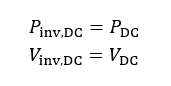# Array Bias (MPP & off-MPP operation)

This function finds the array’s operating point at the array’s nominal (maximum power point) voltage and current bias conditions. Should the MPP fall outside these ranges, the following off-MPP tracking behavior is invoked in an attempt to maintain inverter operation. The inverter operation is divided into 12 zones in the voltage-power space, as show in Figure 41.

The concept of “zone transition” is an artifact of the simulation model, and does not necessarily reflect the transitions of the inverter’s software finite state machine in response to array stimulus. PlantPredict calculates the array bias (voltage & current) at maximum power to determine the “initial zone”. If it happens to be in Zone 6, the MPPT operating window, no further action is taken, and the array bias is set for the current simulation time step.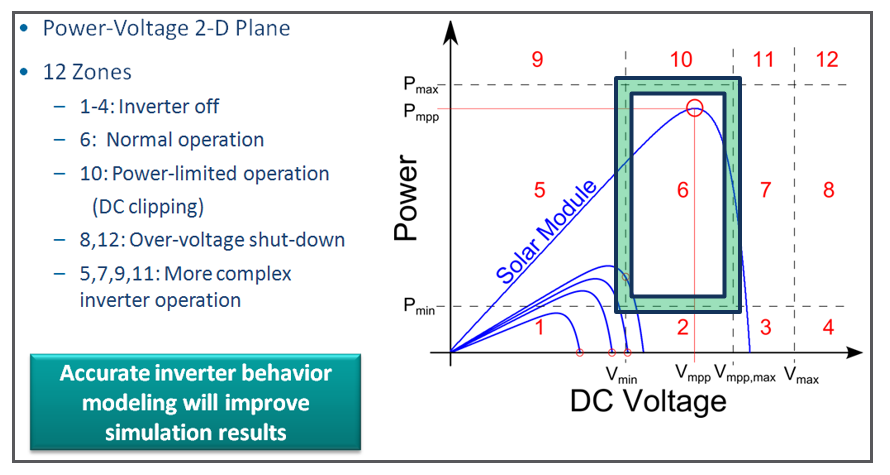### Figure 41. Inverter Operating Window in the Power-Voltage Space, showing 12 Zones of Operation; the Y-axis is AC power

If the array maximum power point falls outside of Zone 6, then PlantPredict seeks to raise or lower the voltage (operate off-MPPT) in order to reach one of the zone boundaries on the green rectangle, specifically the intersections of 5 & 6, 10 & 6, 7 & 6. If the PV curve does not intersect the rectangle, then no power is generated: the inverter is effectively shut off.

## Inputs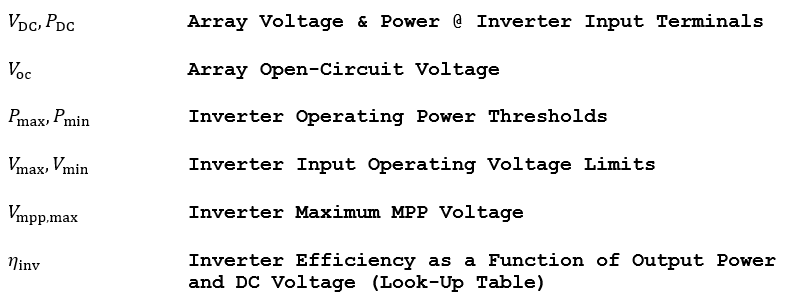## Outputs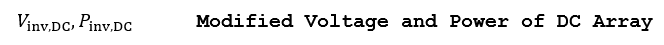## Algorithm

Assume that the inverter is presented with a DC Field biased at VDC to generate DC power, PDC. Verify if the power point falls in one of the following “zones”, and if necessary, modify the array bias, and recheck to see if the power point has changed “zones”. When instructed to check for a zone transition, the following steps should be executed again, with the output set and the procedure exited only if the current “zone” is the same as the “zone” found in the previous iteration.

Zones 1-3: Under-power operation, Pinv,DC < Pmin :

The inverter output is set to zero power. The inverter is shut-off because not enough power can be extracted from the DC Field to operate the inverter’s power electronics. The array remains biased at open-circuit: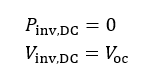Zone 4: Under-power operation & over-voltage operation, Pinv,DC < Pmin & Vinv,DCVmax :

The inverter output is set to zero power. The inverter is shut-off because not enough power can be extracted from the array to operate the inverter’s power electronics. The DC Field is disconnected: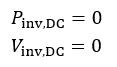Zone 5: Under-voltage operation, Vinv,DCVmaxv :

The inverter has trouble operating because it may not be able to maintain required output voltage due to low input voltage. It will attempt to decrease the current and raise the voltage to reach Vmin; however, if this is not possible, it will set the output power to zero: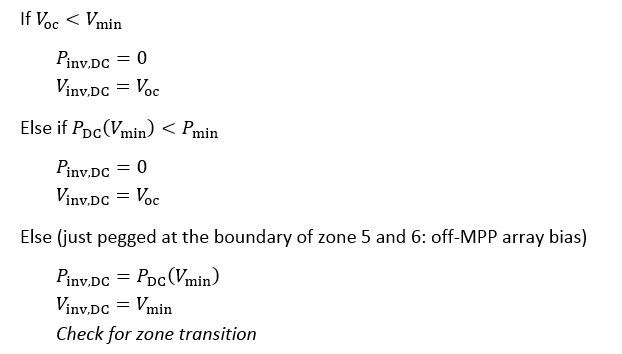Zone 8: Severe over-voltage operation, Vinv,DC > Vmax :

The inverter shuts down and disconnects the DC Field due to over-voltage: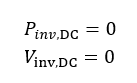Zone 7: Marginal over-voltage operation, Vinv,DC > Vmpp,max :

Increase the current such that voltage drops to Vmpp,max. The operating point slides up the knee of the I(V) curve. Find the new power at Vmpp,max (off-MPP array bias):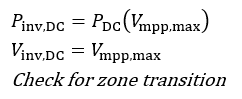Zone 10: Over-power operation Pinv,DC > ηinvPmax :

This is the classic “inverter clipping” case. Raise the inverter voltage in order to reduce the current, such that Pinv,DC = Iinv X Vinv = Pmax. The operating point slides down the knee of the I(V) curve (off-MPP bias):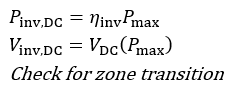Zones 11,12: Over-power operation, Pinv,DC > ηinvPmax & Vinv,DC > Vmpp,max :

This is an extreme over-power and over voltage case. The inverter disconnects the DC Field.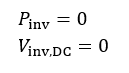Zone 6: Normal operation (fall through case where the maximum power point of the I(V) curve resides in the normal operating window):